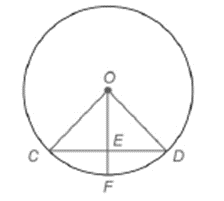Chapter 6.3, Problem 1E### Elementary Geometry for College St...

6th Edition
Daniel C. Alexander + 1 other
ISBN: 9781285195698

#### Solutions

Chapter
Section### Elementary Geometry for College St...

6th Edition
Daniel C. Alexander + 1 other
ISBN: 9781285195698
Textbook Problem
6 views

# Note: Exercises preceded by an asterisk are of a more challenging nature. G i v e n :               ⊙ O     w i t h O E ¯ ⊥ C D ¯                                                             C D = O C F i n d :                     m C F ⌢To determine

To find:

To find mCF^.

Explanation

Given that O with OE¯CD¯ and CD=OC

Consider the following figure,

From the above figure shows that OC=OD. Since radius of O is same for all points on circle.

From the given condition CD=OC=OD

Therefore, ΔOCD is equilateral triangle. Therefore, C=COD=D=60

Let us take ΔOCE,ΔOED

Also, OEC=OED=90. Since OE¯CD¯.

Theorem:

If a line is drawn through center of a circle perpendicular to a chord then it bisects the chord and its arc

### Still sussing out bartleby?

Check out a sample textbook solution.

See a sample solution

#### The Solution to Your Study Problems

Bartleby provides explanations to thousands of textbook problems written by our experts, many with advanced degrees!

Get Started

#### Find more solutions based on key concepts